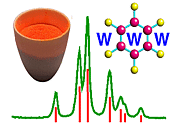Scattering of X-rays by a 1-Dimensional Chain of Atoms or Molecules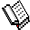Course Material Index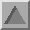Section Index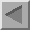Previous Page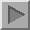Next Page

Scattering of X-rays by a 1-Dimensional Chain of Atoms or Molecules

Moving just slightly closer to reality, we next consider the consequences of X-ray scattering by a chain of atoms or molecules; the situation is idealised in the following diagram (the experiment is again difficult to imagine ever being performed, though perhaps not so impossible as the single electron experiment):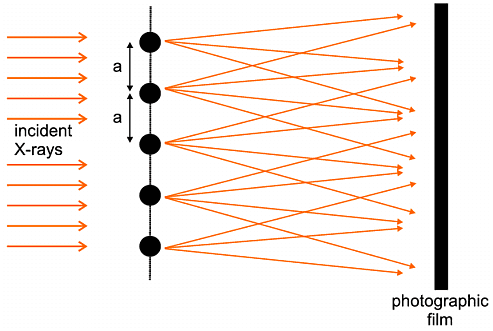The key aspect of this case is that there is a repeating unit (be it an atom or a molecule) and that the unit repeats regularly with a separation, a. It turns out when we mathematically sum up the scattering contributions from all these repeating atoms/molecules one has to take into account that only one of these atoms/molecules can be placed at the space origin, the others being displaced from the origin by a, 2a, 3a, 4a and so on. This results in a scattering formula as follows:
 F(S)  = Σn fne2π i S.rn = f e2π i S.r1 + f e2π i S.(r1 + a ) + f e2π i S.(r1 + 2a) + ....
We can sum such a series and square the result to obtain an expression for the intensity, I, observed on the photographic film (the expression is squared because the intensity is always proportional to the square of an amplitude):
 I =  f 2 {sin N π a.S / sin π a.S}2
The formula has two terms: f 2 which is the scattering from the repeating atom/molecule and the part in brackets which represents the interference (be it destructive or constructive) between the total number, N, of atoms/molecules. We should first consider how this "interference function" changes as we increase the number of atoms/molecules - this is shown below: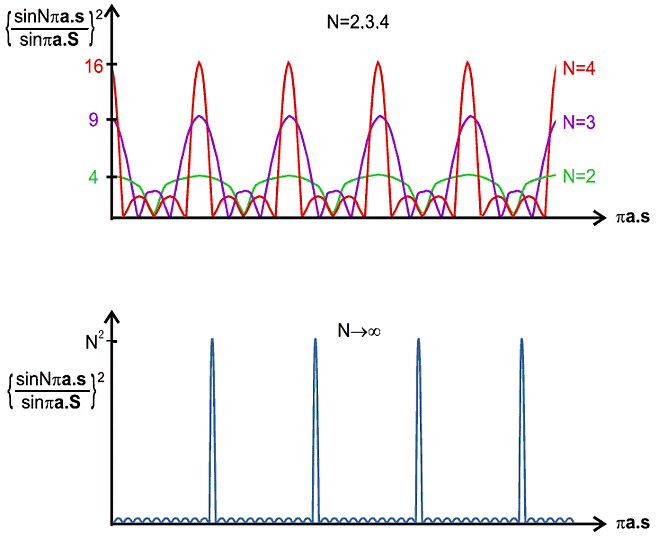The characteristics of this interference function are very interesting. For small N the pattern is somewhat "lumpy" but as N increases to very high values the repeating peaks become sharper and the background becomes lower and flatter; indeed this function begins to acquire some of the features we would recognise in a diffraction pattern; in fact we only have to multiply this function by f 2 to obtain the intensity (as in the first equation) which is in effect a formula for the diffraction pattern, as illustrated below: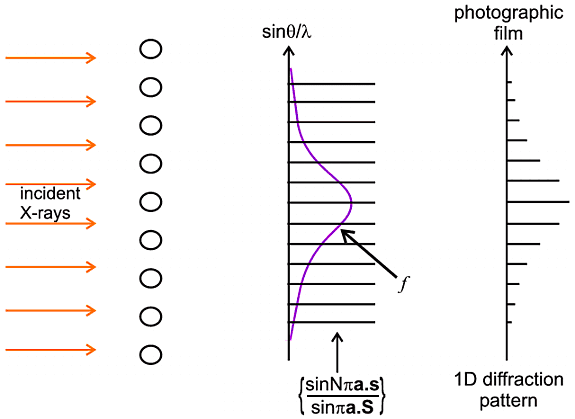Even though this is a somewhat primitive case, it holds some object lessons for later:

• if the number of atom/molecule repeats (N) is small then the diffraction pattern peaks are broad;
• the diffraction pattern becomes sharper as the number of atom/molecule repeats (N) increases;
• the final diffraction pattern is a convolution of two effects: the scattering factor of the repeating (atomic or molecular) unit and the interference resulting from scattering by all the repeating units.Course Material IndexSection IndexPrevious PageNext Page
 © Copyright 1997-2006.  Birkbeck College, University of London. Author(s): Paul Barnes Simon Jacques Martin Vickers